# Samacheer Kalvi 12th Maths Solutions Chapter 5 Two Dimensional Analytical Geometry – II Ex 5.4

## Tamilnadu Samacheer Kalvi 12th Maths Solutions Chapter 5 Two Dimensional Analytical Geometry – II Ex 5.4

Question 1.
Find the equations of the two tangents that can be drawn from (5, 2) to the ellipse 2x2 + 7y2 = 14 .
Solution:
2x2 + 7y2 = 14
(÷ by 14) ⇒ $$\frac{x^{2}}{7}+\frac{y^{2}}{2}$$ = 1
comparing this equation with $$\frac{x^{2}}{a^{2}}+\frac{y^{2}}{b^{2}}$$ = 1
we get a2 = 7 and b2 = 2
The equation of tangent to the above ellipse will be of the form
y = mx + $$\sqrt{a^{2} m^{2}+b^{2}}$$ ⇒ y = mx + $$\sqrt{7 m^{2}+2}$$
Here the tangents are drawn from the point (5, 2)
⇒ 2 = 5m + $$\sqrt{7 m^{2}+2}$$ ⇒ 2 – 5m = $$\sqrt{7 m^{2}+2}$$
Squaring on both sides we get
(2 – 5m)2 = 7m2 + 2
25m2 + 4 – 20m – 7m2 – 2 = 0
18m2 – 20m + 2 = 0
(÷ by 2) ⇒ 9m2 – 10m + 1 = 0
(9m – 1) (m – 1) = 0
‘ m = 1 (or) m = 1/9
When m = 1, the equation of tangent is
y = x + 3 or x – y + 3 = 0
When m = $$\frac{1}{9}$$ the equation of tangent is 9
y = $$=\frac{x}{9}+\sqrt{\frac{7}{81}+2}$$ (i.e.) y = $$\frac{x}{9}+\frac{13}{9}$$
9y = x + 13 or x – 9y + 13 = 0

Question 2.
Find the equations of tangents to the hyperbola $$\frac{x^{2}}{16}-\frac{y^{2}}{64}$$ = 1 which are parallel to 10x – 3y + 9 = 0.
Solution:
$$\frac{x^{2}}{16}-\frac{y^{2}}{64}$$ = 1
Here a2 = 16 and b2 = 64
The equation of tangents will be of the form y = mx ± $$\sqrt{a^{2} m^{2}-b^{2}}$$
(i.e.,) y = mx ± $$\sqrt{16 m^{2}-64}$$
Where ‘m’ is the slope of the tangent.
Here we are given that the tangents are parallel to 10x – 3y + 9 = 0
So slope of tangents will be equatl to slope of the given line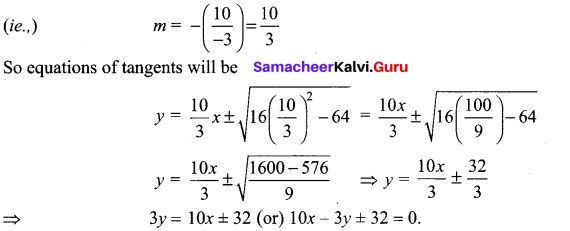Question 3.
Show that the line x – y + 4 = 0 is a tangent to the ellipse x2 + 3y2 = 12. Also find the coordinates of the point of contact.
Solution:
The given ellipse is x2 + 3y2 = 12
(÷ by 12) ⇒ $$\frac{x^{2}}{12}+\frac{y^{2}}{4}$$ = 1
(ie.,) Here a2 = 12 and b2 = 4
The given line is x – y + 4 = 0
(ie.,) y = x + 4
Comparing this line with y = mx + c
We get m = 1 and c = 4
The condition for the line y = mx + c
To be a tangent to the ellipse $$\frac{x^{2}}{a^{2}}+\frac{y^{2}}{b^{2}}$$ = 1 is c2 = a2m2 + b2
LHS = c2 = 42 = 16
RHS: a2m2 + b2 = 12( 1 )2 + 4 = 16
LHS = RHS The given line is a tangent to the ellipse. Also the point of contact is
$$\left(\frac{-a^{2} m}{c}, \frac{b^{2}}{c}\right)=\left[-\left(\frac{12(1)}{4}\right), \frac{4}{4}\right]$$ (i.e.,) (-3, 1)

Question 4.
Find the equation of the tangent to the parabola y2 = 16x perpendicular to 2x + 2y + 3 =0
Solution:
y2 =16x
Comparing this equation with y2 = 4ax
we get 4a = 16 ⇒ a = 4
The equation of tangent to the parabola y2 = 16x will be of the form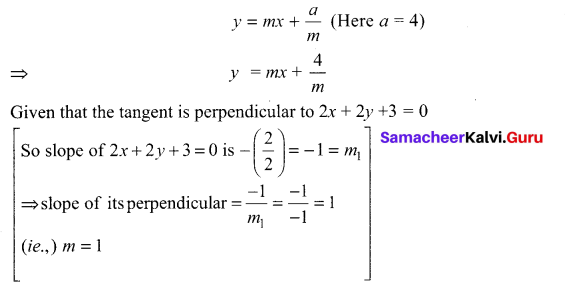So m = 1
⇒ The equation of tangent will be y = 1(x) + $$\frac{4}{1}$$ (i.e.,) y = x + 4
(or) x – y + 4 = 0

Question 5.
Find the equation of the tangent at t = 2 to the parabola y2 = 8x. (Hint: use parametric form)
Solution:
y2 = 8x .
Comparing this equation with y2 = 4ax
we get 4a = 8 ⇒ a = 2
Now, the parametric form for y2 = 4ax is x = at2, y = 2at
Here a = 2 and t = 2
⇒ x = 2(2)2 = 8 and y = 2(2) (2) = 8
So the point is (8, 8)
Now eqution of tangent to y2 = 4 ax at (x1, y1) is yy1 = 2a(x + x1)
Here (x1, y1) = (8, 8) and a = 2
So equation of tangent is y(8) = 2(2) (x + 8)
(ie.,) 8y = 4 (x + 8)
(÷ by 4) ⇒ 2y = x + 8 ⇒ x – 2y + 8 = 0
Aliter
The equation of tangent to the parabola y2 = 4ax at ‘t’ is
yt = x + at2
Here t = 2 and a = 2
So equation of tangent is
(i.e.,) y(2) = x + 2(2)2
2y = x + 8 ⇒ x – 2y + 8 = 0

Question 6.
Find the equations of the tangent and normal to hyperbola 12x2 – 9y2 = 108 at θ = $$\frac{\pi}{3}$$ .
(Hint: Use parametric form)
Solution:
12x2 – 9y2 = 108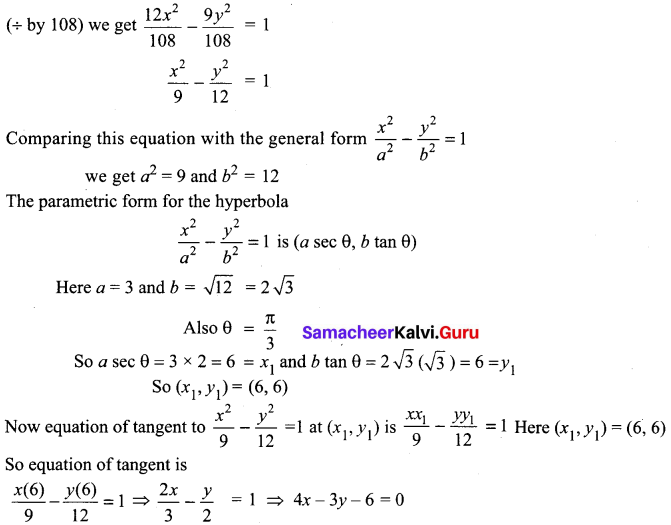Normal is a line perpendicular to tangent
So equation of normal will be of the form 3x + 4y + k = 0
The normal is drawn at (6, 6)
⇒ 18 + 24 + k = 0 ⇒ k = – 42
So equation of normal is 3x + 4y – 42 = 0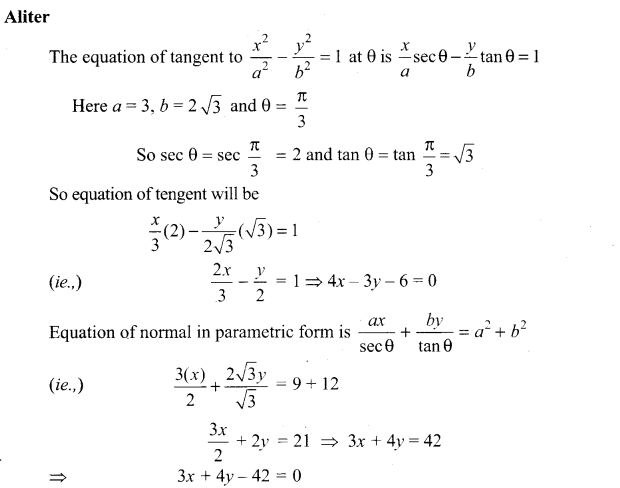Question 7.
Prove that the point of intersection of the tangents at ‘t1‘ and t2’ on the parabola y2 = 4ax is [at1 t2, a (t1 + t2)].
Solution:
The equation of tangent to y2 = 4ax at ‘t’ is given by yt = x + at2
So equation of tangent at ‘t1‘ is yt1 = x + at12
and equation of tangent at ‘t2‘ is yt2 = x + at22
To find the point of intersection we have to solve the two equations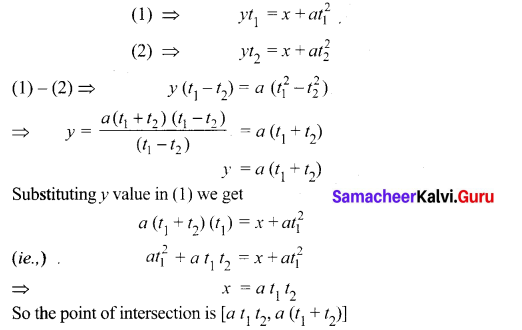Question 8.
If the normal at the point ‘t1‘ on the parabola y2 = 4ax meets the parabola again at the point ‘t2‘, then prove that t2 = $$-\left(t_{1}+\frac{2}{t_{1}}\right)$$
Solution:
Equation of normal to y2 =4 at’ t’ is y + xt = 2at + at3.
So equation of normal at ‘t1’ is y + xt1 = 2at1 + at13
The normal meets the parabola y2 = 4ax at ‘t2’ (ie.,) at (at22, 2at2)
⇒ 2at2 + at1t22 = 2at1 + at13
So 2a(t2 – t1) = at13 – at1t22
⇒ 2a(t2 – t1) = at1(t12 – t22)
⇒ 2(t2 – t1) = t1(t1 + t2)(t1 – t2)
⇒ 2= -t1(t1 + t2)
⇒ t1 + t2 = $$\frac{-2}{t_{1}}$$
⇒ t2 = $$-t_{1}-\frac{2}{t_{1}}=-\left(t_{1}+\frac{2}{t_{1}}\right)$$

### Samacheer Kalvi 12th Maths Solutions Chapter 5 Two Dimensional Analytical Geometry – II Ex 5.4 Additional Questions

Question 1.
Find the equations of the tangent and normal to the parabolas : x2 + 2x – 4y + 4 = 0 at (0, 1)
Solution: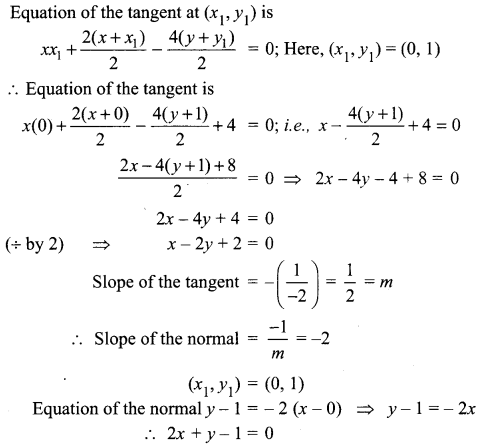Question 2.
Find the equations of the tangent and normal to the parabola y2 = 8x at t = $$\frac{1}{2}$$
Solution: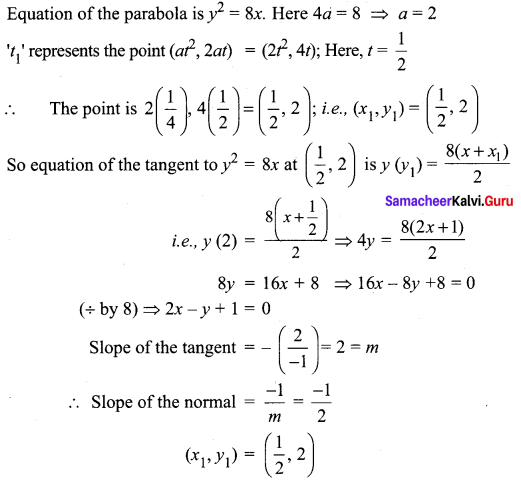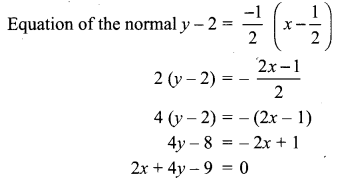∴ Equation of the tangent is 2x – y + 1 = 0 and equation of the normal is 2x + 4y – 9 = 0

Question 3.
Find the equations of the tangents: to the parabola y2 = 6x, parallel to 3x – 2y + 5 = 0
Solution: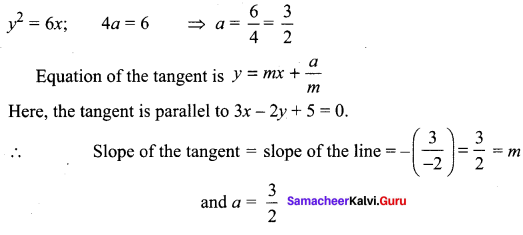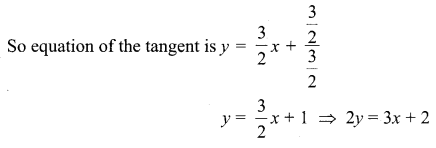∴ Equation of the tangent is 3x – 2y + 2 = 0

Question 4.
Find the equations of the tangents: to the parabola 4x2 – y2 = 64 Which are parallel to 10x – 3y + 9 = 0.
Solution: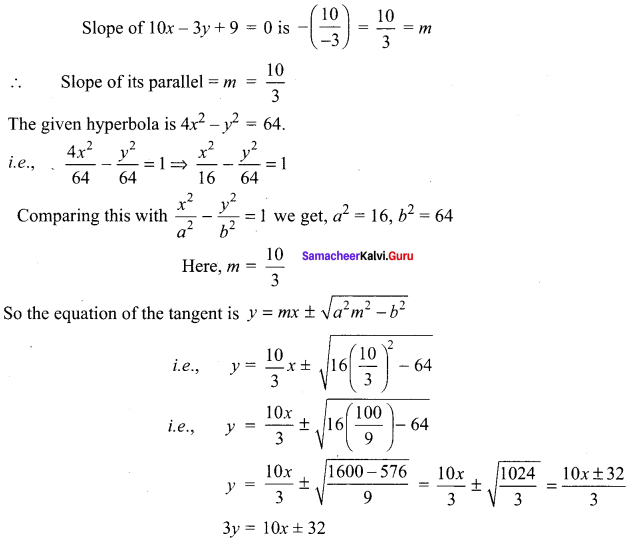Question 5.
Find the equation of the tangent from point (2, -3) to the parabola y2 = 4x.
Solution:
y2 = 4x
Equation of the tangent to the parabola will be of the form y = $$m x+\frac{1}{m}$$
The tangents pass through (2, -3)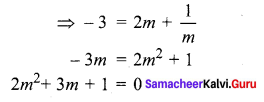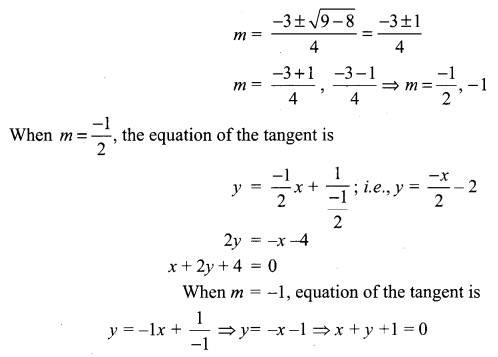The two tangents drawn from (2, -3) are x + y + 1 = 0, x + 2y + 4 = 0Question 6.
Find the equation of the tangents from the point (2, -3) to the parabola 2x2 – 3y2 = 6
Solution:
2x2 – 3y2 = 6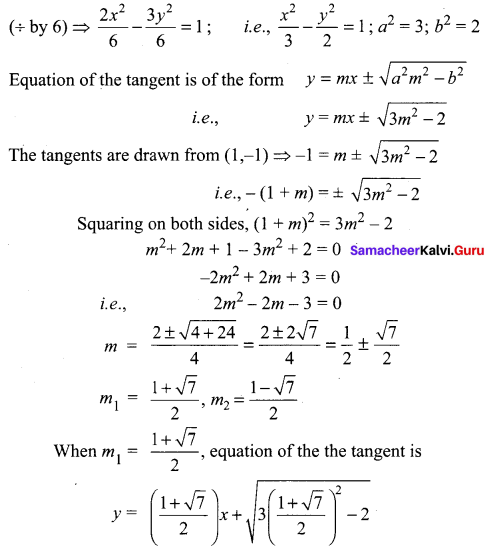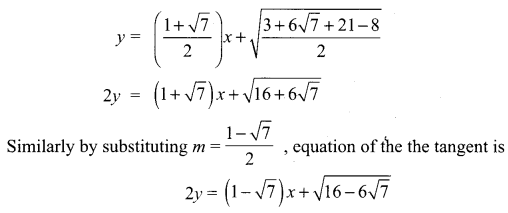Question 7.
Prove that the line 5x + 12y = 9 touches the hyperbola x2 – 9y2 = 9 and find the point of contact.
Solution: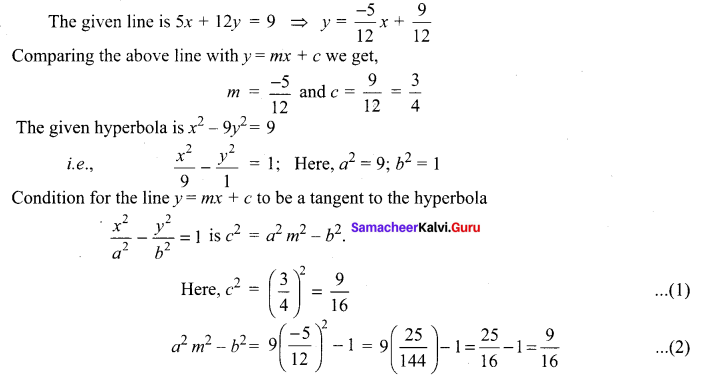(1) = (2) ⇒ The given line is a tangent to the curve i.e., the given line touches the curve. To find the point of contact we have to solve the line and the curve.
The given line 5x + 12y = 9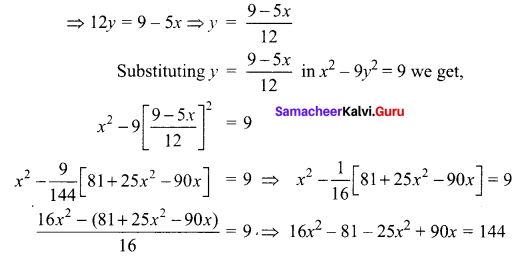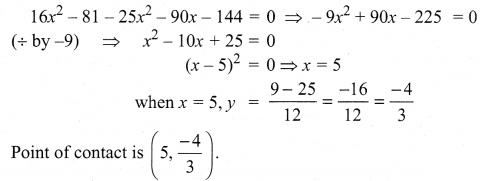Question 8.
Show that the line x – y + 4 = 0 is a tangent to the ellipse x2 + 3y2 = 12. Find the co-ordinates of the point of contact.
Solution:
The given ellipse is x2 + 3y2 = 12
Given line is x – y + 4 = 0 ⇒ y = x + 4
Here, m = 1 and c = 4
The condition for the line y = mx + c to be a tangent to the ellipse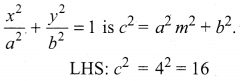RHS: a2 m2 + b = 12(1) + 4 = 16
LHS: RHS ⇒ the given lines is a tangent to the ellipse.